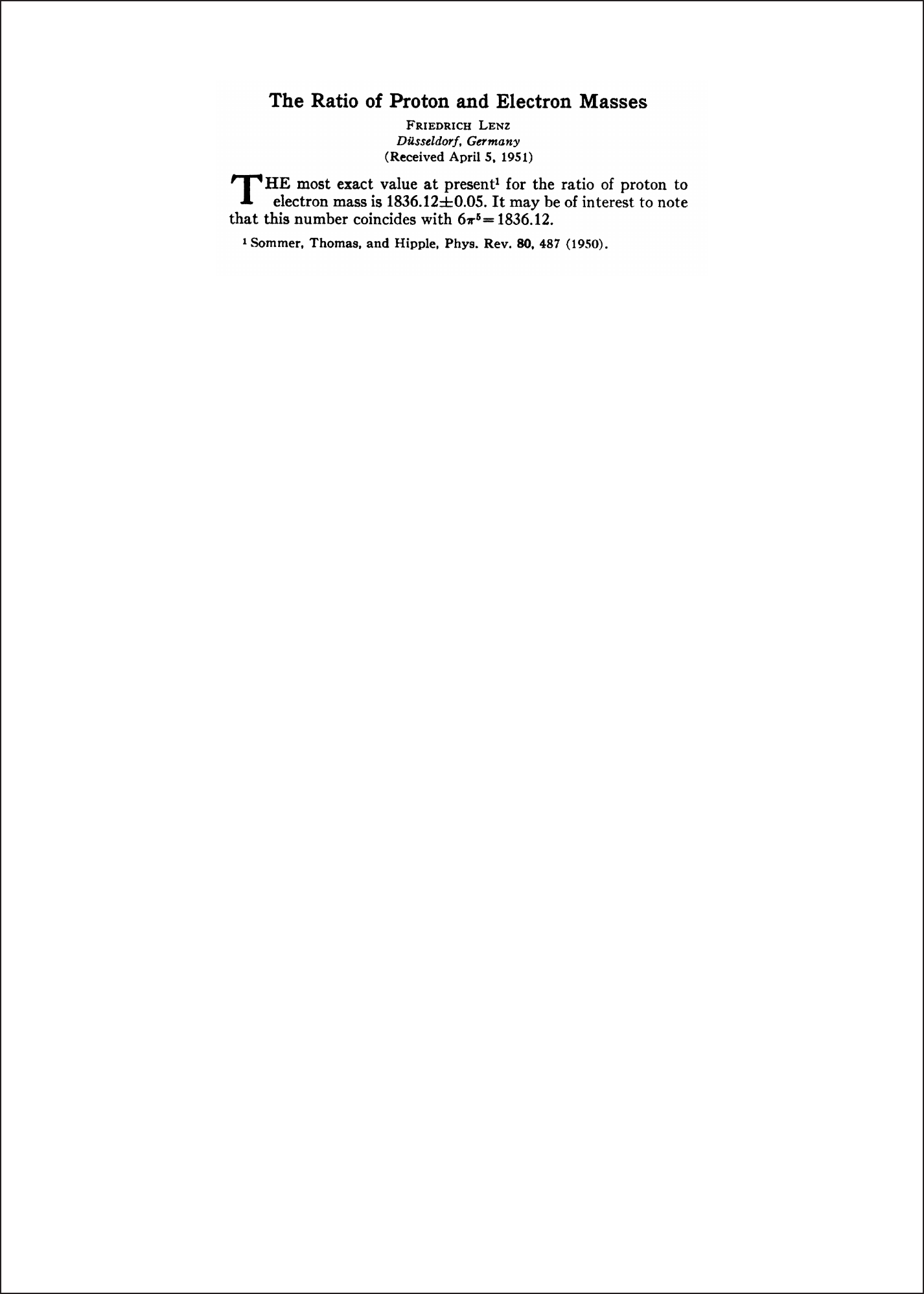This paper by Friedrich Lenz is a really interesting piece of physi...
The author, Friedrich Lenz is another famous "Lenz" in the history ...
As of today, the experimental value of the proton-to-electron mass ...As of today, the experimental value of the proton-to-electron mass ratio is $\mu = m_p/m_e =$ 1836.15267343(11) which still coincides with $6 \pi^5$ to 5 significant figures. Considering the tremendous number of possible values of short polynomial functions of well known constants, this is at most somewhat surprising. The difference in values also makes it nearly certain that the these numbers being close in value is coincidental, rather than reflecting any fundamental relationship. The author, Friedrich Lenz is another famous "Lenz" in the history of physics - not to be confused with Heinrich Lenz of induced currents and Wilhelm Lenz of the conserved vector. Love this! An entire Phys. Rev. Letter that should have been a tweet. In case anyone is wondering--I was--with currently known values of the fundamental constants, Lenz's formula is correct to about one part in 50,000. [How close was Lenz?](https://docs.google.com/spreadsheets/d/1aEO4XqSP_6N_adbWdt294EtmM0IMSR-y0I_nDv4wVLE/edit?usp=sharing) This paper by Friedrich Lenz is a really interesting piece of physics history. In 1951, Lenz wrote a very short Letter to the Editor of Physical Review noting that the value of the ratio $m_p/m_e$ could be expressed to all significant figures of the time by $6π^5$. Lenz's note is the shortest article ever published in Physical Review: 27 words, 1 number, 1 equation, and 1 reference. This type of coincidence is not uncommon in physics and usually leads to a deeper question: is this a signal of some undiscovered theory of reality or just a mere fluke? For this particular case, Ariel Amir, Mikhail Lemeshko, and Tadashi Tokieda calculated in 2016 using a simple model that for an expression built up from well-known mathematical constants the a priori probability of getting a 5-digit number like 1836.1 is only 1.2% (original paper [here](https://arxiv.org/pdf/1603.00299.pdf))